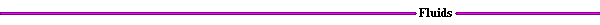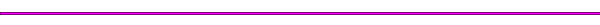Intake FlowFigure 2.  Intake Diagram

Due to the constant opening and closing of the valves, the cross sectional area through which the flow passes through changes. This area is referred to as the effective area. This area is defined as:

Ae = Ac (q ) Cd(q)
Where:
Ac = curtain area
Cd = discharge coefficient

The curtain area, Ac, can be defined in several ways. A simple definition of the curtain area is given by:

Ac = 3.14159*Dv Lv

Where:
Dv = diameter of the valve
Lv = lift

The lift and the discharge coefficient both vary with the crank angle. The discharge coefficient is determined experimentally. This coefficient accounts for the real gas flow effects. The discharge coefficient decreases slightly with lift since the jet fills less of the reference curtain area as it transforms from an attached jet to a separated free jet. A representative value of the discharge coefficient is Cd = 0.6.

The lift and Cd are determined from experiment. If the lift and Cd profiles are known , the effective area can be found as a function of the crank angle. The following applet, Intake Effective Area Applet , determines the effective area as defined above.